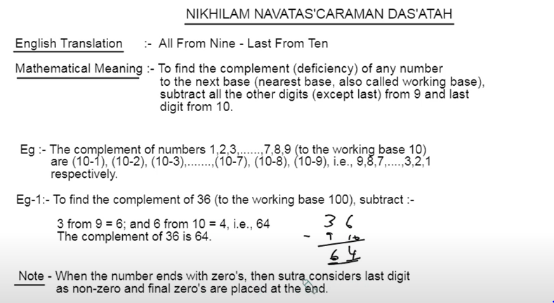Search
• Mamta Rupesh

# Vedic Maths Tricks along with PDF

Vedic Maths relies on a mixture of simple tricks and formulas to make maths easy. The ancient Vedic Sutras form the basis of Vedic Maths which are a set of memorized solutions to basic mathematical problems. I have already explained the meaning, benefits, and Vedic Maths in the previous blogs, videos, and podcasts. In addition, you will also find a lot of useful tips to improve your knowledge on this subject. So if you are interested then keep reading further.

Here you will be able to see a range of practical problems where Vedic Maths Tricks are applied to solve sums easily. When compared to conventional maths, Vedic Maths has its interesting tricks to calculate answers faster.

Moreover, I'm going to present you with examples here.

1- To calculate “Squares of Numbers Ending in 5”

2- To Calculate “Subtraction of Numbers from Base”

3- To Calculate “Multiplication by 9,99,999,...”

4- To Calculate "Magic with 11"

5- To Calculate "Tables upto 19"

If you guess the Vedic Maths tricks are only for the fancy numbers then it's a BIG NO, We have tricks for any given numbers which can be used in our day-to-day activities, school curriculum, and of course in competitive exams and any other place where maths is put to work.

Before that, You can check our website, In our roadmap, we have the Vedic Maths Tricks course which covers a few interesting methods along with pdfs.

All our courses will contain a pdf below every chapter.

Over 5000+ students have purchased this course and have been benefited. We have various topics where the Vedic Maths Tricks are used, lets see what are they:-

We can solve any type of Arithmetic, Algebra, Geometry, Trigonometry Etc...

Eg:- Starting from-

Subtraction

Multiplication by 5, 9, 11, 12, 13…,

Vertically and Crosswise method

Base method,

Magic Division in one line

Squares of numbers ending in 5

Random Squares

Cubes in one line

Interesting Square roots and Cube roots,....

Not only Arithmetic, even Algebraic topics like Quadratic equations, simultaneous equations, linear equations, algebraic multiplication, etc.. are covered.

I wouldn't say all the topics are easy, there are a few complicated ones too, but mostly it's easy and interesting. Especially, we need to have good practice over it.

You might think, without any proof why should we come to a conclusion that Vedic Maths Tricks is easier, so for that, here I'm going to show you the examples.

## To calculate “Squares of Numbers Ending in 5”

### The Sutra name is Ekadhikena Purvena:-

English Translation:- One more than the previous one.

Mathematical Meaning:- To obtain the next number, add one to the previous one. (4+1=5).

Eg:- Find 25²?

We need to multiply 2 x 3 (i.e, 2+1= 3 or take directly its next consecutive number), then write 5² (i.e., 25)

(2x3) 5²## To Calculate “Subtraction of Numbers from Base”

### The Sutra name is Nikhilam Navatashcaramam Dashatah:-

English Translation:- All from Nine, Last from Ten.

Mathematical Meaning:- To find the complement (deficiency) of any number to its next base (nearest base also called the working base).

Subtract all the other digits (except last) from 9 and the last digit from 10.

Eg:- Find the complement of 64?

100 - 64, so we need to subtract 6 from 9 and the last digit 4 from 10. Therefore, the answer is 36.## To Calculate “Multiplication by 9,99,999,...”

### The Sutra name is Ekanyunena Purvena:-

English Translation:- One Less than the Previous One.

Mathematical Meaning:- One number less than the previous number. (45-1=44)

Eg:- Multiply 67 by 99.

67 x 99 = (67-1) (100-67) = 6633 is the answer.## To Calculate ''Magic with 11''

### Eg:- Multiply 24 by 11.

Multiplying any number with 11,111,...are very easy and the formula is very simple.

get the given numbers outside, add both and write in between, that will be the final answer.

check the below example.

24 x 11

Write 2 and 4 as it is in both the corners and the sum of 2 and 4(2+4) i.e. 6 in between.## To Calculate ''Tables upto 19''

Firstly, we need to know Tables from 2-9, then it's easy to calculate tables upto 99 too.

Eg-1:- 3 x 6 = 18 13 x 6 = 78 (6+1=7, take 8 from 18 as it is)

Eg-2:- 4 x 8 = 32 14 x 8 = 112 (8+3=11, take 2 from 32 as it is)

Eg-3:- 9 x 6 = 54 19 x 6 = 114 (6+5=11, take 4 as it is)

To get the answer, you need to add just the factor (of the corresponding table) to the ten’s place digit of the corresponding product. The one’s digit remains as it is.## Conclusion

Maths is considered to be one of the most difficult and tedious subjects for students. It is common to see children spending hours on a single math problem, struggling to find the answer. With Vedic Maths, the time spent on maths problems can be reduced significantly. Vedic Maths tricks and techniques are easy to learn and will help to solve math problems a breeze.

So we saw a few basic Vedic Maths Tricks and the rest of the topics are covered under the courses. Vedic Maths is a subject that has been gaining popularity over the years. The courses provided by us are applicable for students from grades 3-12, students writing competitive exams, parents, and teachers. They are designed to equip you with the necessary skills for life and school math problems.

You can Learn, Teach and Grow.

By now you would have got the confidence of solving maths easily using Vedic Tricks right, so just stay focused and get started today and become a Maths Genius Tomorrow.

Remember this: You Can Do It! In fact, You Must! Don't waste another second thinking about it or worrying about it. Just get started!